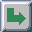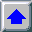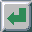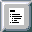Next: Photometry Up: Photometry and Radiometry Previous: Light

Radiometry is the science of measuring light in any portion of the spectrum. Therefore, the color is not important to the radiometry.

Light is radiant energy. Electromagnetic radiation transports energy through space. A broadband source, like the Sun, emits the energy throughout most of the spectrum, while, on the other hand, single-wavelength laser emits radiation only at one specific wavelength.

We can define spectral radiant energy, which is the amount of radiant energy per unit wavelength interval at wavelength, as:Radiant flux is then defined as:where Q is radiant energy, and t is time. Spectral radiant flux is defined as. Radiant flux density is the radiant flux per unit area at a point on the surface. There are two possibilities. The flux can be arriving at the surface (radiant flux density is then called irradiance):And the flux can be leaving the surface (radiant flux density is then referred to as radiant exitance):There are also spectral forms of radiant flux densities,and.

If we think of a ray of light arriving at or leaving a point on a surface in a given direction, then radiance is simply an infinitesimal amount of radiant flux contained in this ray. Actually the ray should be an infinitesimally narrow cone with its apex at a point on a surface. The cone has a differential solid anglethat is measured in steradians. Of course, a ray intersecs the surface at angle. Therefore a projected areainstead of the area dA should be used. The definition of radiance is then:Unlike radiant flux density, the definition of radiance does not distinguish between flux arriving at or leaving the surface. Spectral radiance, as radiance per unit wavelength interval at wavelengthis also defined.Next: Photometry Up: Photometry and Radiometry Previous: Light

matkovic@cg.tuwien.ac.at